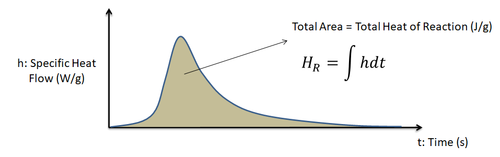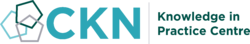The CKN Knowledge in Practice Centre is in the early stages of content creation and currently focuses on the theme of thermal management.
We appreciate any feedback or content suggestions/requests using the links below

# Heat of reaction - A114

Heat of reaction
Foundational knowledge articleDocument Type Article
Document Identifier 114
Themes
Relevant Class

Material

Tags
Prerequisites

## Introduction

The polymerization process for thermoset resins is an exothermic reaction generating heat. Measured in energy/mass, the total heat of reaction represents this thermal energy released by a thermoset polymer during its curing process.

## Scope

This page discusses the material property of the heat of reaction. The property definition, its usage in process modelling, and measurement are briefly discussed.

## Significance

In composites processing of thermoset resin systems, the heat of reaction is an important parameter for cure kinetics modelling. It is involved in determining the resin's degree of cure, and the heat generation term of the thermal management heat equation.

The total heat of reaction is the measure of the exothermic heat release during the thermoset polymer curing reaction. Management of this heat release is important in ensuring a proper curing of the thermoset matrix, where large sudden temperature spikes (exotherms) are to be avoided as they can lead to the thermal degradation of the resin.

## Prerequisites

Recommended documents to review before, or in parallel with this document:

## Definition

The polymerization process for thermoset resins is an exothermic reaction generating heat. Measured in energy/mass, the total heat of reaction $$H_R$$ represents this thermal energy released by a thermoset polymer during its curing process.

As illustrated below, the total heat of reaction of the resin system is the area under a differential scanning calorimetry (DSC) obtained heat flow curve.An example of a heat flow curve obtained from differential scanning calorimetry (DSC) measurement of a resin sample starting from an unreacted state. The total heat of reaction is the area under the heat flow curve.

### Terminology and Symbol Notation

#### Terminology

In most composite processing literature, the term heat of reaction is used. However, it should be noted that the chemistry term for the energy released from an exothermic reaction process is enthalpy of reaction $$\Delta H$$ or $$\Delta H_r$$, and when normalized by mass - described as specific enthalpy.

#### Symbol Notation

In most composite processing related literature, the heat of reaction is represented as $$H_R$$, $$H_r$$, or $$H_T$$.

Symbol notation for heat of reaction
Common reported forms $$H_R$$, $$H_r$$, $$H_T$$
Other reported forms $$\Delta H$$, $$\Delta H_r$$ (enthalpy - traditional chemistry notation)

### Units

The units of the heat of reaction are given in energy unit per mass.

$$H_R=\frac{Energy\, unit}{Mass\, unit}$$

SI Units US Customary Units
Base units J/kg BTU/lb
Other common forms J/g

### Typical Property Values

The amount of exothermic energy released is polymerization and cross-linking reaction dependent. As a result, every unique thermoset resin system has the potential to release a certain and different amount of total energy.

#### Example of different total heat of reaction values for different thermoset resin systems

For popular composite thermoset matrix polymers such as polyesters and epoxies, typical total heat of reaction values range from 300-600 J/g.

### Usage of Heat of Reaction

#### Degree of cure

Heat of reaction is used as an index measure to determine the degree of cure of the resin system. The degree of cure ($$x$$) is defined as the remaining heat that can be further released compared to the total amount of heat energy release possible for the resin system. It is used to quantify the extent of the thermosetting curing (crosslinking) reaction.

$$x=1-\frac{H_{res}}{H_R}$$

Where,

$$H_{res}=$$ Residual heat of reaction (unreacted portion) [J/kg]

$$H_R=$$ Total heat of reaction of the resin system [J/kg]

#### Heat generation term

The heat of reaction is an important parameter for modelling the resin heat generation$\dot{Q_r}=\frac{d\ x}{dt}H_RV_r\rho_r$

Where,

$$\dot{Q_r}=$$ Resin heat generation rate per volume of resin [J/s·m3]

$$\frac{d\ x}{dt}=$$ Rate change of degree of cure [1/s]

$$H_R=$$ Heat of reaction [J/kg]

$$V_r=$$ Volume fraction of resin

$$\rho_r=$$ Resin density [kg/m3]

## Measurement

### Differential Scanning Calorimetry (DSC)

The heat of reaction during polymerization is measured using Differential Scanning Calorimetry (DSC). The DSC measures the amount of heat energy released during reaction for a small resin sample. It is one of the common thermal tests performed to characterize a resin system and to determine the extent of resin cure in a sample. Sampling in the DSC requires only a small amount of sample, 10-30mg.

As illustrated earlier in the definition section, the total heat of reaction of the resin system is the area under the DSC obtained heat flow curve.

## Related pages

Introduction to Composites Articles
Foundational Knowledge Articles
Foundational Knowledge Method Documents
Foundational Knowledge Worked Examples
Systems Knowledge Articles
Systems Knowledge Method Documents
Systems Knowledge Worked Examples
Systems Catalogue Articles
Systems Catalogue Objects – Material
Systems Catalogue Objects – Shape
Systems Catalogue Objects – Tooling and consumables
Systems Catalogue Objects – Equipment
Practice Documents
Case Studies
Perspectives Articles## Welcome

Welcome to the CKN Knowledge in Practice Centre (KPC). The KPC is a resource for learning and applying scientific knowledge to the practice of composites manufacturing. As you navigate around the KPC, refer back to the information on this right-hand pane as a resource for understanding the intricacies of composites processing and why the KPC is laid out in the way that it is. The following video explains the KPC approach:

## Understanding Composites Processing

The Knowledge in Practice Centre (KPC) is centered around a structured method of thinking about composite material manufacturing. From the top down, the heirarchy consists of:

The way that the material, shape, tooling & consumables and equipment (abbreviated as MSTE) interact with each other during a process step is critical to the outcome of the manufacturing step, and ultimately critical to the quality of the finished part. The interactions between MSTE during a process step can be numerous and complex, but the Knowledge in Practice Centre aims to make you aware of these interactions, understand how one parameter affects another, and understand how to analyze the problem using a systems based approach. Using this approach, the factory can then be developed with a complete understanding and control of all interactions.

## Interrelationship of Function, Shape, Material & Process

Design for manufacturing is critical to ensuring the producibility of a part. Trouble arises when it is considered too late or not at all in the design process. Conversely, process design (controlling the interactions between shape, material, tooling & consumables and equipment to achieve a desired outcome) must always consider the shape and material of the part. Ashby has developed and popularized the approach linking design (function) to the choice of material and shape, which influence the process selected and vice versa, as shown below:

Within the Knowledge in Practice Centre the same methodology is applied but the process is more fully defined by also explicitly calling out the equipment and tooling & consumables. Note that in common usage, a process which consists of many steps can be arbitrarily defined by just one step, e.g. "spray-up". Though convenient, this can be misleading.

## Workflows

The KPC's Practice and Case Study volumes consist of three types of workflows:

• Development - Analyzing the interactions between MSTE in the process steps to make decisions on processing parameters and understanding how the process steps and factory cells fit within the factory.
• Troubleshooting - Guiding you to possible causes of processing issues affecting either cost, rate or quality and directing you to the most appropriate development workflow to improve the process
• Optimization - An expansion on the development workflows where a larger number of options are considered to achieve the best mixture of cost, rate & quality for your application.